Latest Banking jobs   »

# Reasoning Ability Quiz For NABARD Grade A 2022- 6th September

Directions (1-3): Study the following information and answer the given answers.
Seven people A, C, E, P, R, S and O are studying in the same standard. Their scores in Mathematics are different but not necessarily in the same order. A scores more than C who scores more than E. R scores more than only O. At least one person scores less than E but more than R. P’s score is not the highest. S scores just less than P.

Q1. Whose score is the highest?
(a) A
(b) C
(c) S
(d) E
(e) None of the above

Q2. Who among the following scores more than E?
(a) P
(b) S
(c) R
(d) O
(e) None of the above

Q3. Who among the following scores just more than P?
(a) A
(b) C
(c) R
(d) O
(e) E

Directions (4-5): Study the information given below and answer the questions based on it.
Six persons A, B, C, D, E, and F are having different weights but not necessarily in the same order. C is heavier than F. E is only lighter than one person. A is heavier than D. F is heavier than D. B is heavier than A. The number of people heavier than A is the same as lighter than C. A is heavier than C.

Q4. How many persons are lighter than A?
(a) None
(b) One
(c) Two
(d) Three
(e) More than three

Q5. Who among the following is the lightest person?
(a) A
(b) B
(c) F
(d) C
(e) D

Directions (6-8): Study the following information carefully and answer the questions accordingly.
Point X is 30m east of point Y and 20m north of point W. Point T is 10m north of point S and point U is 20m east of point T. Point Q is 20m west of point P. Point M is 30m north of point N and 40m west of point O. Point V is 40m north of point U and 30m east of point W. Point R is 30m south of point Q and 40m west of point S. Point P is 20m south of point O.

Q6. Point Y is in which direction of point Q?
(a) West
(b) South
(c) North
(d) South-West
(e) None of these

Q7. What is the distance between M and W?
(a) 60m
(b) 70m
(c) 50m
(d) 40m
(e) Can’t be determined

Q8. Point T is in which direction of point Q?
(a) South-East
(b) North-West
(c) North-East
(d) South-West
(e) Can’t be determined

Directions (9-10): Study the following information carefully and answer the questions accordingly.
Point W is 5m to the south of point V which is 8m to the west of point U. Point T is 5m to the south of Point U. Point P is 4m to the north of Point Q. Point Q is 5m to the west of Point R. Point S is 4m to the north of Point R. Point T is 6m to the east of Point S.

Q9. What is the direction of Point W with respect to Point Q?
(a) North
(b) North-east
(c) South-west
(d) North-west
(e) None of these

Q10. What is the total distance between Point W and Point R?
(a) 26m
(b) 20m
(c) 29m
(d) 28m
(e) None of these

Directions (11-15): Study the information carefully and answer the following questions.
Eight people i.e. A, B, C, D, E, F, G and H sit in a square-shaped table but not necessarily in the same order. Four people sit at the four corners of the table and face outside the table. Four people sit at the middle of the four sides and face inside the table. Two people sit between A and H who sits at the corner. B sits second to the left of H. Only one person sits between B and D. G sits second to the left of D. C sits second to the right of E. F does not sit adjacent to D and G.

Q11. Four of the following five are alike in a certain way and hence from a group. Find the one who doesn’t belong to that group?
(a) C
(b) H
(c) D
(d) G
(e) B

Q12. How many people sit between F and G when counted from right of G?
(a) Four
(b) Three
(c) One
(d) Two
(e) None of these

Q13. Which statement is true?
(a) D sits adjacent to G.
(b) Two people sit between A and C.
(c) E faces F
(d) H faces inside the table
(e) None of these

Q14. If all the people sit according to the English alphabetical order from A in clockwise direction, then how many people remains unchanged? (Excluding A)
(a) Two
(b) One
(c) Three
(d) Four
(e) None of these

Q15. Who sits fourth to the left of D?
(a) C
(b) H
(c) F
(d) G
(e) None of these

Solutions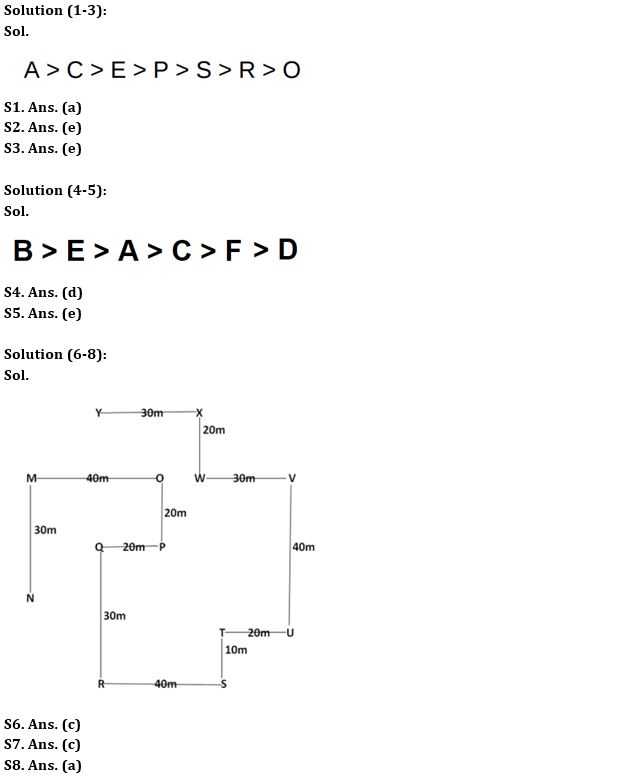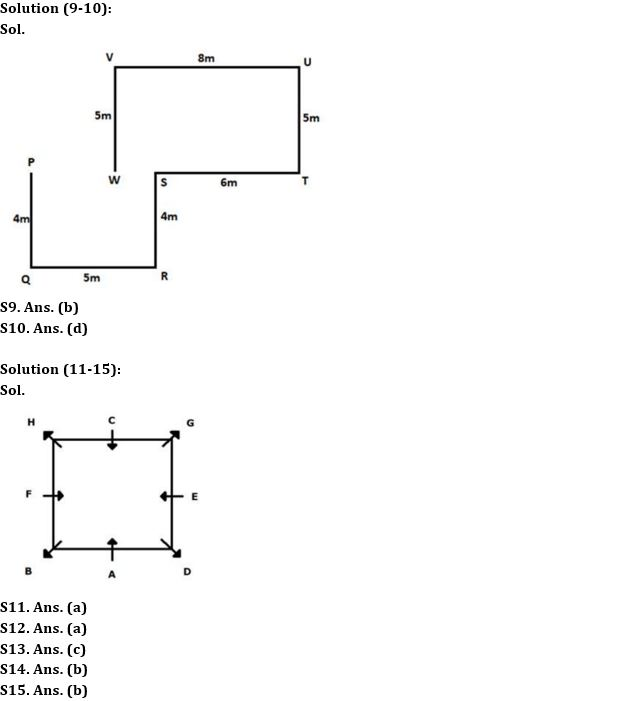#### Congratulations!Download Hindu Review of October 2021: Free PDFIncorrect details? Fill the form again here

Download Hindu Review of October 2021: Free PDF

Thank You, Your details have been submitted we will get back to you.
•General Awareness Quiz Series 2022: 4th ...
•World Animal Welfare Day 2022, Theme, Hi...
•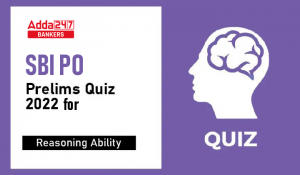Reasoning Ability Quiz For SBI PO Prelim...
•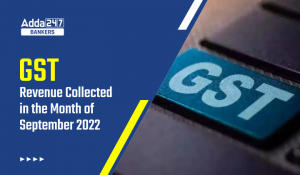GST revenue collected in the month of Se...
•Reasoning Ability Quiz For FCI Phase I 2...
•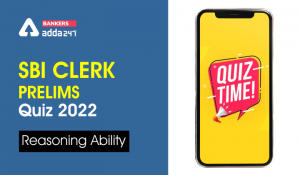Reasoning Ability Quiz For SBI Clerk Pre...